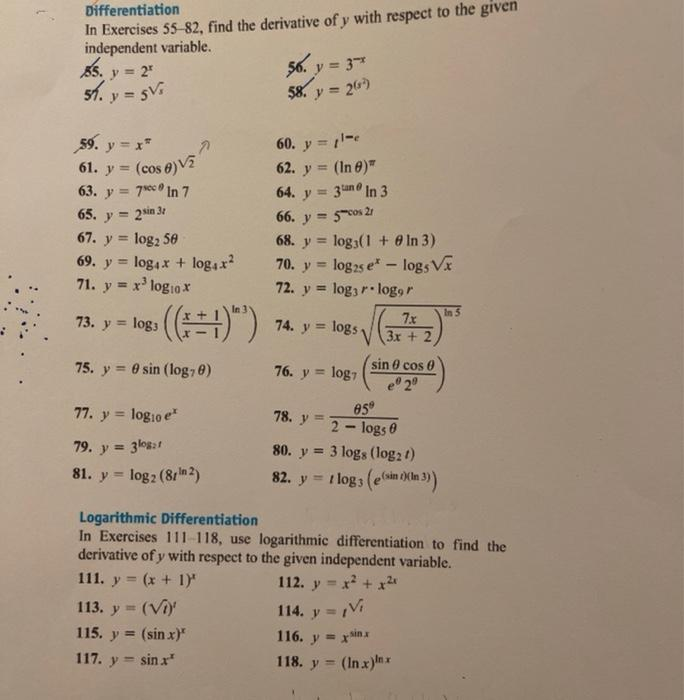### Create an Account

Already have account?

### Forgot Your Password ?

Home / Questions / Differentiation In Exercises 55 82, find the derivative of y with respect to the given ind...

# Differentiation In Exercises 55 82, find the derivative of y with respect to the given independent variable. y = 2 56. y = 3 sh. y = ghi 58. y = 267 64. y 59. y = * 61. y = (cos 6) V2 63. y = 70 in 7

Differentiation In Exercises 55 82, find the derivative of y with respect to the given independent variable. y = 2 56. y = 3 sh. y = ghi 58. y = 267 64. y 59. y = * 61. y = (cos 6) V2 63. y = 70 in 7 65. y = 67. y = log2 5e 69. y = log x + logar? 71. y = 2sin 31 60. y = -6 62. y = (In)" 3un ® In 3 66. y = 5s 21 68. y = log3(1 + In 3) 70. y = log as e* - logs VX 72. log3 r. loger 7.6 V (3x + 2 x logo In 5 73. y = log2 (==1)") 74. y = logs 75. y = 8 sin (log70) sin cos 76. y = log? e20 78. y 05 77. y = logio e' 79. y = 3los: 1 81. y = log2 (8rin2) 2 - logs 80. y = 3 logs (log21) 82. y = 1 logs (esan och )) Logarithmic Differentiation In Exercises 111-118, use logarithmic differentiation to find the derivative of y with respect to the given independent variable. 111. y = (x + 1)" 112. y = x2 + x2 113. y = (VO 115. y = (sin x)" 116. y = xin 117. y = sinx" 118. y = (In xnx 114. y =
pls. 60, 62,63,65,69,71,72,73,75,77,78,79,80,81
111,113,115,117,116,114Apr 29 2021 View more View Less

#### Answer (Solved)Subscribe To Get Solution